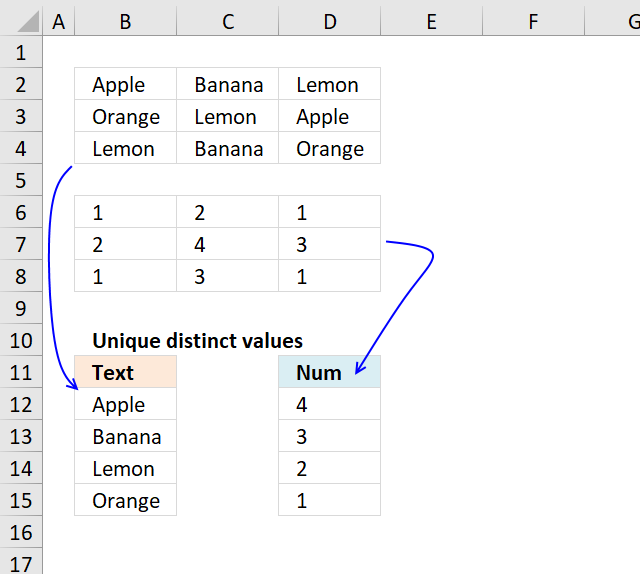Author: Oscar Cronquist Article last updated on November 12, 2018Question: I have cell values spanning over several columns and I want to create a unique list from that range. How?

Thanks to Eero, who contributed the original array formula!Unique distinct text values from range tbl_text, array formula in B13:

=INDEX(tbl_text, MIN(IF(COUNTIF(\$B\$12:B12, \$B\$2:\$D\$4)=0, ROW(\$B\$2:\$D\$4)-MIN(ROW(\$B\$2:\$D\$4))+1)), MATCH(0, COUNTIF(\$B\$12:B12, INDEX(\$B\$2:\$D\$4, MIN(IF(COUNTIF(\$B\$12:B12, \$B\$2:\$D\$4)=0, ROW(\$B\$2:\$D\$4)-MIN(ROW(\$B\$2:\$D\$4))+1)), , 1)), 0), 1)

Recommended articles:

Extract unique distinct values A to Z from a range and ignore blanks

This is an answer to a question in this blog post: Extract a unique distinct list sorted from A-Z from […]

Extract a unique distinct list sorted from A-Z from range

The image above shows an array formula in cell B8 that extracts unique distinct values sorted alphabetically from cell range […]

Extract unique distinct values from a multi-column cell range

Question: I have cell values spanning over several columns and I want to create a unique list from that range. […]

Extract duplicates from a range

The following array formula in cell B11 extracts duplicates from cell range B3:E8, only one instance of each duplicate is […]

### How to enter an array formula

1. Double press with left mouse button on cell B13
2. Copy and paste above formula to cell B13
3. Press and hold CTRL + SHIFT simultaneously
4. Press Enter once
5. Release all keys

Your formula now looks like this: {=array_formula}

Don't enter these characters yourself, they appear automatically when you do above steps.

Recommended article

A beginners guide to Excel array formulas

Array formulas allows you to do advanced calculations not possible with regular formulas.

### How to copy array formula

Copy cell B13 and paste it down as far as necessary.

Unique distinct numbers from range tbl_num, array formula in D13:

=LARGE(IF(COUNTIF(\$D\$12:D12, tbl_num)=0, tbl_num, ""), 1) + CTRL + SHIFT + ENTER

Copy cell D13 and paste it down as far as necessary.

### Explaining array formula in cell B13

The array formula has two parts. One part returns row numbers and the other part returns column numbers. Let us begin with the first part, returning row numbers.

Step 1 - Find new unique distinct text values

=INDEX(tbl_text, MIN(IF(COUNTIF(\$B\$12:B12, tbl_text)=0, ROW(tbl_text)-MIN(ROW(tbl_text))+1)), MATCH(0, COUNTIF(\$B\$12:B12, INDEX(tbl_text, MIN(IF(COUNTIF(\$B\$12:B12, tbl_text)=0, ROW(tbl_text)-MIN(ROW(tbl_text))+1)), , 1)), 0), 1)

COUNTIF(range,criteria) - Counts the number of cells within a range that meet the given condition

COUNTIF(\$B\$12:B12, tbl_text)=0

becomes

COUNTIF("Text", {"Apple","Banana","Lemon";"Orange","Lemon","Apple";"Lemon","Banana","Orange"})=0

becomes

{0,0,0;0,0,0;0,0,0}=0

becomes

{TRUE,TRUE,TRUE;TRUE,TRUE,TRUE;TRUE,TRUE,TRUE}

How to use the COUNTIF function

Counts the number of cells that meet a specific condition.

Step 2 - Convert boolean array to row numbers

=INDEX(tbl_text, MIN(IF(COUNTIF(\$B\$12:B12, tbl_text)=0, ROW(tbl_text)-MIN(ROW(tbl_text))+1)), MATCH(0, COUNTIF(\$B\$12:B12, INDEX(tbl_text, MIN(IF(COUNTIF(\$B\$12:B12, tbl_text)=0, ROW(tbl_text)-MIN(ROW(tbl_text))+1)), , 1)), 0), 1)

IF(logical_test,[value_if:true],[value_if_false]) checks whether a condition is met, and returns one value if TRUE, and another value if FALSE

IF(COUNTIF(\$B\$12:B12, tbl_text)=0, ROW(tbl_text)-MIN(ROW(tbl_text))+1)

becomes

IF({TRUE,TRUE,TRUE;TRUE,TRUE,TRUE;TRUE,TRUE,TRUE}, {2;3;4}-MIN({2;3;4})+1)

becomes

IF({TRUE,TRUE,TRUE;TRUE,TRUE,TRUE;TRUE,TRUE,TRUE}, {2;3;4}-MIN({2;3;4})+1)

becomes

IF({TRUE,TRUE,TRUE;TRUE,TRUE,TRUE;TRUE,TRUE,TRUE}, {2;3;4}-2+1)

becomes

IF({TRUE,TRUE,TRUE;TRUE,TRUE,TRUE;TRUE,TRUE,TRUE}, {1;2;3}) and returns {1,1,1;2,2,2;3,3,3}

How to use the IF function

Checks if a logical expression is met. Returns a specific value if TRUE and another specific value if FALSE.

Step 3 - Extract smallest value in array

=INDEX(tbl_text, MIN(IF(COUNTIF(\$B\$12:B12, tbl_text)=0, ROW(tbl_text)-MIN(ROW(tbl_text))+1)), MATCH(0, COUNTIF(\$B\$12:B12, INDEX(tbl_text, MIN(IF(COUNTIF(\$B\$12:B12, tbl_text)=0, ROW(tbl_text)-MIN(ROW(tbl_text))+1)), , 1)), 0), 1)

MIN(number1,[number2])
Returns the smallest number in a set of values. Ignores logical values and text

MIN(IF(COUNTIF(\$B\$12:B12, tbl_text)=0, ROW(tbl_text)-MIN(ROW(tbl_text))+1))

becomes

MIN({1,1,1;2,2,2;3,3,3}) and returns 1.

Step 4 - Part two, identify array values in current row

=INDEX(tbl_text, MIN(IF(COUNTIF(\$B\$12:B12, tbl_text)=0, ROW(tbl_text)-MIN(ROW(tbl_text))+1)), MATCH(0, COUNTIF(\$B\$12:B12, INDEX(tbl_text, MIN(IF(COUNTIF(\$B\$12:B12, tbl_text)=0, ROW(tbl_text)-MIN(ROW(tbl_text))+1)), , 1)), 0), 1)

INDEX(array,row_num,[column_num]) returns a value or reference of the cell at the intersection of a particular row and column, in a given range

INDEX(tbl_text, MIN(IF(COUNTIF(\$B\$12:B12, tbl_text)=0, ROW(tbl_text)-MIN(ROW(tbl_text))+1)), , 1))

becomes

INDEX(tbl_text, MIN({1,1,1;2,2,2;3,3,3}), , 1))

becomes

INDEX(tbl_text, 1, , 1)) returns array {"Apple", "Banana", "Lemon"}

How to use the INDEX function

Gets a value in a specific cell range based on a row and column number.

Step 5 - Find new unique distinct text values in current row

=INDEX(tbl_text, MIN(IF(COUNTIF(\$B\$12:B12, tbl_text)=0, ROW(tbl_text)-MIN(ROW(tbl_text))+1)), MATCH(0, COUNTIF(\$B\$12:B12, INDEX(tbl_text, MIN(IF(COUNTIF(\$B\$12:B12, tbl_text)=0, ROW(tbl_text)-MIN(ROW(tbl_text))+1)), , 1)), 0), 1)

COUNTIF(range,criteria) counts the number of cells within a range that meet the given condition

COUNTIF(\$B\$12:B12, INDEX(tbl_text, MIN(IF(COUNTIF(\$B\$12:B12, tbl_text)=0, ROW(tbl_text)-MIN(ROW(tbl_text))+1)), , 1))

becomes

COUNTIF("Text", {"Apple", "Banana", "Lemon"}) and returns {0,0,0}

Step 6 - Find a new unique distinct text value in current row

=INDEX(tbl_text, MIN(IF(COUNTIF(\$B\$12:B12, tbl_text)=0, ROW(tbl_text)-MIN(ROW(tbl_text))+1)), MATCH(0, COUNTIF(\$B\$12:B12, INDEX(tbl_text, MIN(IF(COUNTIF(\$B\$12:B12, tbl_text)=0, ROW(tbl_text)-MIN(ROW(tbl_text))+1)), , 1)), 0), 1)

MATCH(lookup_value;lookup_array; [match_type]) returns the relative position of an item in an array that matches a specified value

MATCH(0, COUNTIF(\$B\$12:B12, INDEX(tbl_text, MIN(IF(COUNTIF(\$B\$12:B12, tbl_text)=0, ROW(tbl_text)-MIN(ROW(tbl_text))+1)), , 1)), 0)

becomes

MATCH(0, {0,0,0}, 0) returns 1.

How to use the MATCH function

Identify the position of a value in an array.

Step 7 - All together

=INDEX(tbl_text, MIN(IF(COUNTIF(\$B\$12:B12, tbl_text)=0, ROW(tbl_text)-MIN(ROW(tbl_text))+1)), MATCH(0, COUNTIF(\$B\$12:B12, INDEX(tbl_text, MIN(IF(COUNTIF(\$B\$12:B12, tbl_text)=0, ROW(tbl_text)-MIN(ROW(tbl_text))+1)), , 1)), 0), 1)

becomes

=INDEX(tbl_text, 1, 1) returns value "Apple" in cell B13.

### Explaining array formula in cell D13

=LARGE(IF(COUNTIF(\$D\$12:D12, tbl_num)=0, tbl_num, ""), 1)

Step 1 - Remove previously extracted values above current cell with an array with boolean values

=LARGE(IF(COUNTIF(\$D\$12:D12, tbl_num)=0, tbl_num, ""), 1)

COUNTIF(range,criteria) - Counts the number of cells within a range that meet the given condition

COUNTIF(\$D\$12:D12, tbl_num)=0

becomes

{0,0,0;0,0,0;0,0,0}=0

becomes

{TRUE,TRUE,TRUE;TRUE,TRUE,TRUE;TRUE,TRUE,TRUE}

Step 2 - Convert boolean values to numeric values

=LARGE(IF(COUNTIF(\$D\$12:D12, tbl_num)=0, tbl_num, ""), 1)

IF(logical_test;[value_if:true];[value_if_false]) checks whether a condition is met, and returns one value if TRUE, and another value if FALSE

IF(COUNTIF(\$D\$12:D12, tbl_num)=0, tbl_num, "")

becomes

IF({TRUE,TRUE,TRUE;TRUE,TRUE,TRUE;TRUE,TRUE,TRUE}, {1, 2, 1;2, 4, 3;1, 3, 1}, "")

becomes

{1, 2, 1;2, 4, 3;1, 3, 1}

Step 3 - Convert boolean values to numeric values

LARGE(array,k) returns the k-th largest row number in this data set.

=LARGE(IF(COUNTIF(\$D\$12:D12, tbl_num)=0, tbl_num, ""), 1)

becomes

=LARGE({1, 2, 1;2, 4, 3;1, 3, 1}, 1) returns 4 in cell D13.

How to use the SMALL function

The SMALL function lets you extract a number in a cell range based on how small it is compared to the other numbers in the group.